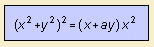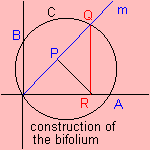# bifolium

## quarticThe bifolium 1) has been studied by Longchamps (1886) and Brocard (1887), and is also named the double folium.

The bifolium can be constructed as follows.
Given a circle C through O, A(0, a) and B(1, 0), and a line m through O that cuts C in Q. Let R be the projection of Q on the x-axis, and P the projection of Q on m.
Then the bifolium is the collection of points P, where m varies in the given circle C.

The bifolium for a and for -a are each other's reflection in the x-axis.

notes

1) In German: Zweiblatt.